LEVEL 8:

39.        The diagram shows a motor, connected to a 240 V supply, driving a water pump.

The ammeter reads 5.0 A.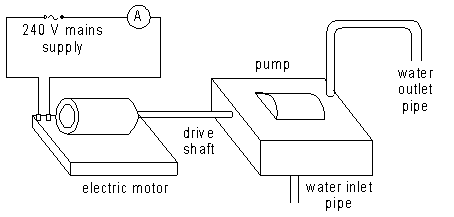(a)      How much charge flows through the motor in one minute? Give the unit.

1 mark

(b)      (i)       What is the resistance of the motor? Give the unit.

.

1 mark

(ii)      What is the power of the motor? Give the unit

.

1 mark

(c)      (i)       The motor and pump together have an efficiency of 50% (0.5).  How much energy is given to the water every second? Give the unit.

1 mark

(ii)      The pump has to raise the water up to a vertical height of 12 m.  What mass of water will the pump raise each second? Give the unit.  The value of g is 10 N/kg.                                             1 mark

40.         Three ways of generating electricity are shown in the table.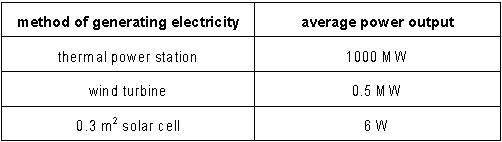A small offshore island with a population of 1000 has yet to be provided with a mains electricity supply.  It is expected that the island will need an average of 250 watts per person, but in winter this may rise to 1000 watts (1 kW) per person.

(a)      Calculate the area of land on the island which would have to be covered by solar cells in order to generate electricity at the same average power as one wind turbine (0.5 MW).  Give the unit.                                           1 mark

(b)      The islanders decided against using solar cells.  They were left with a choice of being connected to the national grid by undersea cable or of having a single wind turbine.  The capital costs of each choice are nearly the same but it costs twice as much to buy a unit of energy from the national grid as to obtain one from the wind turbine.

The islanders chose to be connected by undersea cable to the national grid.

(i)       Use the information above to suggest one disadvantage of the wind turbine which may have influenced their decision.                                            1 mark

(ii)      Suggest one other disadvantage of the wind turbine.                                            1 mark

41.     Stars such as the Sun are formed from vast and diffuse clouds of gas and dust.  The clouds contract to form stars as gravity pulls the gas and dust together.

(a)      As a cloud contracts, it becomes hotter.  Explain why, in terms of the movement of gas molecules in the cloud.                                2 marks

(b)      In the later stages of collapse, the contraction of the cloud is slowed down by a number of factors.  Suggest one factor which would slow down the contraction of the cloud.

1 mark

(c)      What are the main processes by which energy is transferred into space from the hot centre of the collapsed cloud? State where these processes occur.                                  2 marks

(d)      Before nuclear fusion begins, the contracting cloud is very much more difficult to observe than stars which are the same distance away.

Explain why the cloud is difficult to observe.                                          1 mark

(e)      What is the most abundant chemical element in the cloud?

1 mark

(f)       Eventually the temperature at the centre of the cloud becomes high enough for nuclear fusion to begin.  What happens during nuclear fusion?

1 mark

42.      Transformers are always connected to a.c. supplies.  A pupil decides to investigate the effect of connecting a transformer to a d.c. supply.  He sets up the following circuit to try to transform 12 volts from a car battery down to 2 volts.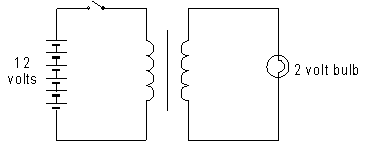(a)      What would you expect to see when the switch is closed?                               1 mark

Explain why this happens                              2 marks

(b)      What would you expect to see when the switch is left closed?                               1 mark

Give a reason for your answer.                               1 mark

(c)      What would you expect to see when the switch is opened?                             1 mark

(d)      In the circuit diagram, the straight line between the coil symbols represents an iron core.

Tick the box to show the purpose of the core in a transformer.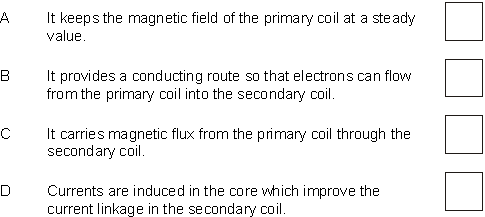1 mark

43.         The graph shows the speed of a ball as it falls from a height and bounces from the floor.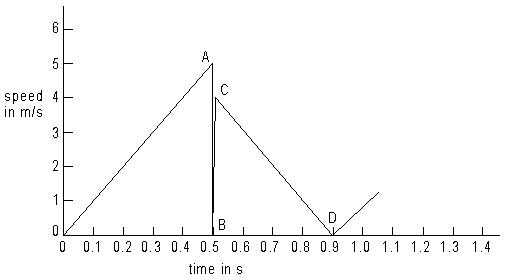(a)  The ball starts to fall and speeds up until it hits the floor.

(i)  For how many seconds does the ball fall before it first hits the floor?

1 mark

(ii)   Calculate the average speed of the ball during its fall.

1 mark

(iii)    Calculate the height above the floor from which the ball was dropped.

1 mark

(b)      (i)     What is happening to the ball in the time between points A and C on the graph?

1 mark

(ii)      In which direction is the ball moving between points C and D?

1 mark

(c)      Calculate how high the ball bounces back up from the floor.

1 mark

(d)      How long after the ball was dropped would you expect it to hit the floor for the second bounce?

1 mark

44.         The diagram shows a regular hexagonal glass prism.

A ray of light passes through the centre of the hexagon.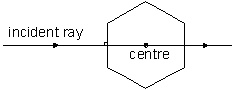(a)      The diagram below shows the same prism in a different position.
The ray of light is aimed at the centre of the hexagon.

Complete the ray as it passes through the prism and show its direction when it emerges.  Use a ruler.

2 marks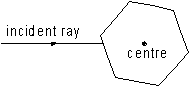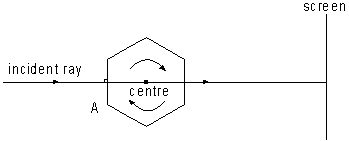(b)      A ray of light is aimed at the centre of the hexagonal prism, which is slowly rotating.    Describe how the spot of light on the screen moves:

(i)       as point A approaches the incident ray;

1 mark

(ii)      as point A crosses the incident ray;

1 mark

(iii)     as the prism continues rotating until it reaches the position shown in part (a) above.                                                                                                                           1 mark

45.      (a)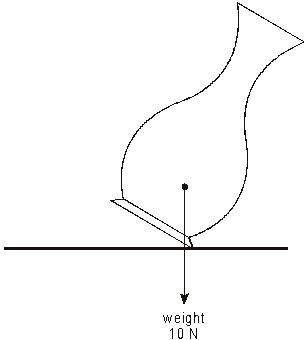A vase is held at an angle as shown in the diagram above. When it is released it falls back on its base.

Explain why, in terms of moments.

1 mark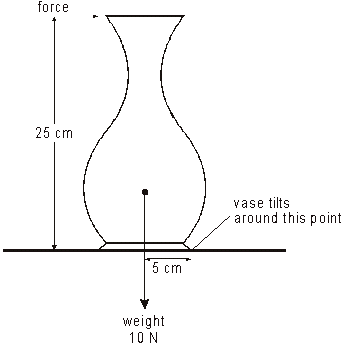(b)      In the diagram, above, the top of the vase is being given a push.
The force is just large enough to make the vase start to tilt.

(i)       Calculate the size of the force.  Show your working and give the correct unit.

3 marks

(ii)      If the base of the vase were wider, a larger force would be needed to make the vase start to tilt. Explain why, in terms of moments.

1 mark

46.      The simplified diagram shows a device which protects people from being electrocuted when using power tools such as hedge cutters. The wire in one part of the circuit is shown darker to make the circuit clearer.

When the armature is attracted towards the soft iron core, the contacts are pushed apart and break the circuit.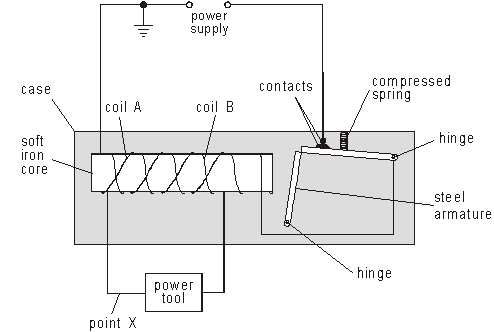(a)      The power tool, coil A and coil B are all in the same series circuit. Coils A and B are wound in opposite directions.

The current in the coils has no magnetic effect on the armature. Explain why.

1 mark

(b)      A gardener accidentally cuts the wire at point X. Current from the power supply starts to flow through the gardener to earth.

What effect will this have on the armature? Explain your answer.

3 marks